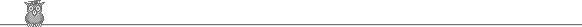### 27th USAMO 1998Problem B2

Show that one can find a finite set of integers of any size such that for any two members the square of their difference divides their product.

Solution

We find inductively a set with n elements satisfying the slightly stronger condition that if a and b are any two elements, then a - b divides both a and b. For n = 2, we may take {1, 2}. Suppose we have a set S for n. Let m be the lowest common multiple (or any multiple) of all the members of S. Now take the set {m + a: a ∈ S} ∪ {m} for n+1. A difference (m + a) - (m + b) = a - b divides a and b, hence also m, and hence m + a and m + b. A difference (m + a) - m = a divides a and m and hence also m + a.